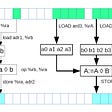# Sparse Systolic Tensor Array for Efﬁcient CNN Hardware Acceleration

Convolutional neural networks can also have accelerated by data path optimization. Early CNN accelerators have implemented Systolic arrays to perform fast and memory-efficient data operations. In this blog, we will discuss these systolic arrays in detail. CNNs are composed of a high number of convolution layers (155 in ResNet152). These convolution operations include a large number of the general matrix to matrix multiplications (GEMM). So optimizing these matrix multiplications can have a larger impact on the time complexity and power consumption of CNNs.

Zhi-Gang Liu et al. have demonstrated a sparse systolic arrays approach to accelerate the 2D filtering in convolution layers. Generally, data sparsity accelerates the CNN because theoretically, zeros in the data reduce the computational complexity and space complexity also. There are three types of data sparsity in matrices:

1. Random sparse: The non-zero elements in the data are randomly distributed. But in this case, every non-zero element needs to be indexed explicitly. So it requires more storage units to store the indices. (Fig. 1 (a))
2. Block sparse: Elements in the whole block are either zero or non-zero. This arrangement is beneficial for hardware design as it doesn’t require indexing every element explicitly. The block of zeros can be indexed with a single index. But this affects the accuracy of CNNs. (Fig. 1 (b))
3. Density bounding block (DBB) sparsity: In this structure, there is a bound on the number of non-zero elements in each block. This arrangement has hardware acceleration benefits and also it won’t affect the accuracy of the CNN.

The authors have proposed a new DBB arrangement called variable density bounding block (VDBB). CNNs have different levels of data sparsity. So with this arrangement of non-zero elements, different sparsity ratios can be achieved.

# Systolic arrays:

GEMM are performed very efficiently with systolic arrays. This systolic array implements local register-to-register operand reuse. It consists of a two-dimensional array of processing elements (PE). Each processing element consists of one multiplier accumulator (MAC) with an INT8 operand. The advanced version of the systolic array is the systolic tensor array (STA) in which the processing element takes the whole matrix (tensor) and matrix of activations as input. Each tensor processing element performs multiple matrix operations. Authors have integrated VDBB in systolic tensor array architecture which can improve energy and area efficiency in a matrix multiplication operation. Generally, structured sparsity in the model optimizes the CNNs to improve the throughput and energy efficiency, and proposed sparsity architecture (VDBB) tackles the problem of a wide range of sparsity levels in real-world CNNs.

References:

1. Zhi-Gang Liu, et al., Sparse Systolic Tensor Array for Efficient CNN Hardware Acceleration, arXiv, 2009.02381. (2020)

2. P. Warden. Why GEMM is at the heart of deep learning. [Online]. Available: https://petewarden.com/2015/04/20/why-gemm-is-at-the-heartof-deep-learning/

--

--

--

## More from VLSI for Neural Networks and Artificial Intelligence

Neural Network Model Deployment on VLSI Devices

## Nimble: Lightweight and Parallel GPU Task Scheduling for Deep Learning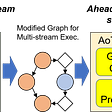## What is Pattern X?## Drunk Guy Conundrum(Loss and Optimization)Lec3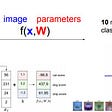## How I Built a Machine Learning Playground with Streamlit## How does Starbucks use machine learning (ML)?## KNN Algorithm: A Practical Implementation Of KNN Algorithm In R## Machine Learning : Supervised and Unsupervised Learning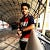## Modern Neural Networks (AlexNet) in the ILSVRC## Instant Neural Graphics Primitives with a Multiresolution Hash Encoding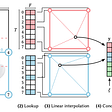## All About Activation Functions in Neural Network## Vector Instructions. Part I